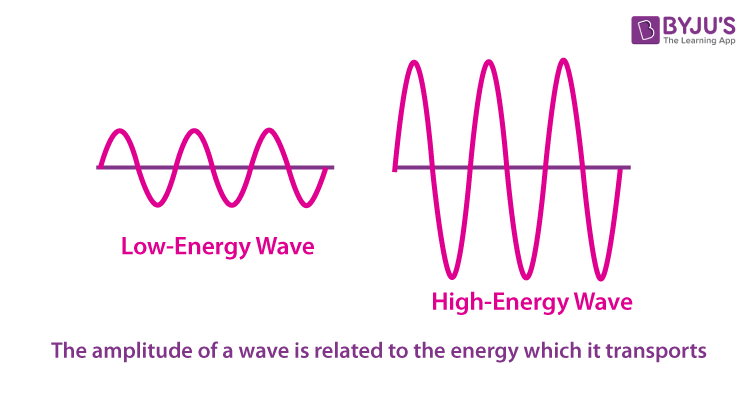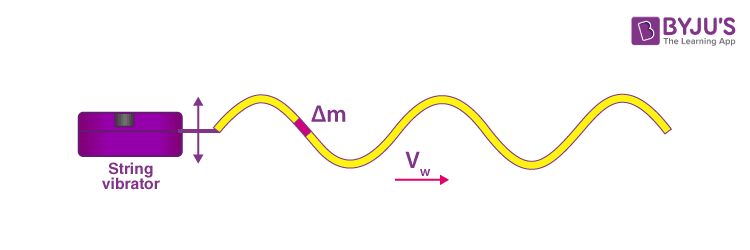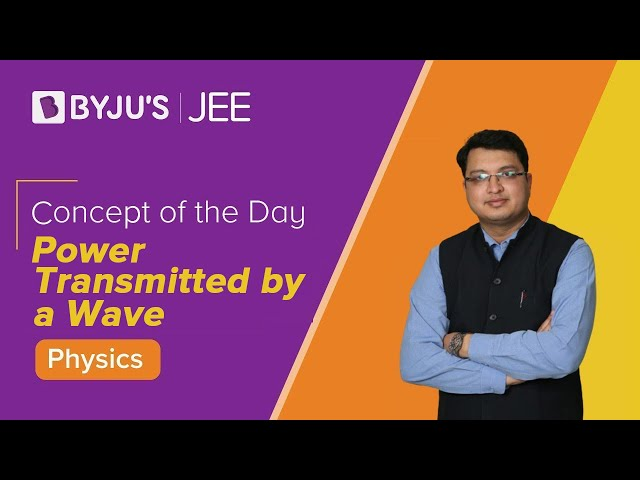# Energy of a Wave

All waves carry energy. The energy of some waves, such as the energy of earthquakes, can be directly observed. Similarly, the churning of the shore by the water waves and the pulverization of nerve cells due to loud sounds are manifestations of wave energy. In this article, we shall be examining the quantitative expression of energy in waves. And, we shall also understand what gives waves their energy and how amplitude and frequency relate to wave energy.

## How is the energy of a wave related to the amplitude?

The amount of energy carried by a wave directly depends on its amplitude. A high amplitude defines a high energy wave. Likewise, a low energy wave is characterized by a low amplitude. To better understand the energy-amplitude relationship, let us consider a slinky. A given slinky is stretched in a horizontal direction, and a transverse pulse is introduced into it by providing the first coil of the slinky an initial amount of displacement. The given displacement is due to the force applied by the person to displace it from rest. The more force imparted to the coil, the more displacement it will exhibit; as a result, the more amplitude it will have. Concluding, we can say that the amplitude of a transverse pulse is directly related to the energy with which the pulse transports through the medium. The energy of the transverse pulse will not change the wavelength, the frequency or the speed of the pulse; it will only affect the amplitude of the pulse.### Wave Energy-Amplitude Relationship

The energy transported by a wave is directly proportional to the square of the amplitude of the wave. This energy-amplitude relationship is mathematically expressed as follows:## Energy of a Wave Formula

To obtain the mathematical expression for the energy of a wave, consider a sinusoidal wave on a string that is produced by a string vibrator, as shown in the figure below:The string vibrator is a device that vibrates a rod up and down. A string of uniform linear mass density is fastened to the rod. The rod oscillates the string, creating a sinusoidal wave. The rod does a certain amount of work on the string generating energy that travels along the string. Consider a mass element of the string with a mass Δm, as shown in the figure. As the energy travels along the string, each mass element of the string is driven up and down at the same frequency as the wave. The mass element of the string has the mass $$\Delta m=\mu \Delta x$$ as the string has a constant linear density.

The total mechanical energy of the wave is the sum of its kinetic energy and potential energy.

The kinetic energy associated with the wave can be represented as:

$$U_{Kinetic}=\frac{1}{4}(\mu A^2\omega ^2\lambda )$$

A is the wave amplitude, ω is the angular frequency of the wave oscillator, λ is the wavelength, and µ is the constant linear density of the string.

The following equation represents the potential energy of the string:

$$U_{Potential}=\frac{1}{4}(\mu A^2\omega ^2\lambda )$$

A is the wave amplitude, ω is the angular frequency of the wave oscillator, λ is the wavelength, and µ is the constant linear density of the string.

Thus, the total energy of the wave is given as:

UTotal = UPotential + UKinetic

$$=\frac{1}{4}(\mu A^2\omega ^2\lambda )+\frac{1}{4}(\mu A^2\omega ^2\lambda )$$

$$=\frac{1}{2}(\mu A^2\omega ^2\lambda )$$

In the video, you will learn to derive the expression for the power transmitted by a wave.## Frequently Asked Questions on Energy of a Wave

### Do all waves carry energy?

Yes, all waves carry energy.

### What is the total energy of a wave?

The potential energy and kinetic energy associated with a given wavelength of the wave are equal. Therefore, the total energy associated with a wavelength is the sum of the potential energy and the kinetic energy.

### How is the energy of the wave related to its amplitude?

The amount of energy carried by a wave directly depends on its amplitude. Thus, a high amplitude defines a high energy wave. Likewise, a low energy wave is characterized by a low amplitude.

### What will happen to the energy of the wave as frequency increases?

The higher the frequency, the more energy carried by the wave.

### What is the Wave Energy-Amplitude Relationship?

The energy transported by a wave is directly proportional to the square of the amplitude of the wave.